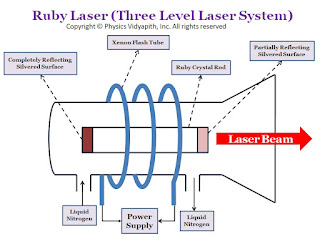Showing posts with label Ray Optics. Show all posts
Showing posts with label Ray Optics. Show all posts

## Refraction of light and its Properties

Definition of Refraction of light→ When a light goes from one medium to another medium then light bends from the path. This bending of the light phenomenon is knowns as the refraction of light.

When light goes from a rarer medium to a denser medium, light bends toward the normal as shown in the figure below: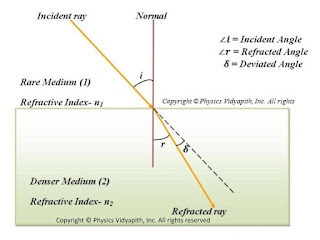Propagation of light from rarer medium to denser medium
When light goes from a denser medium to a rarer medium, the light goes away from normal as shown in the figure below.Propagation of light from denser medium to rarer medium

Properties of refraction of light→

1. The incident ray, normal ray, and refracted ray lie on the same point.
2. According to Snell's law - The ratio of the sine of the incident angle to the sine of the refractive angle is always constant. This constant value is known as the refractive index of the medium.

 $\frac{sin \: i}{sin \: r}=constant(_{1}n_{2})$

$\frac{sin \: i}{sin \: r}= (_{1}n_{2})$

$\frac{sin \: i}{sin \:r}=\frac{n_{2}}{n_{1}}$

Where $_{1}n_{2}$ is the refractive index of medium $(2)$ with respect to medium $(1)$ .

Absolute refractive index→

The ratio of the speed of light in a vacuum to the speed of light in a medium is called the absolute refractive index of the medium.

$refractive\: index(n)=\frac{speed\: of\:light\: in\: vacuum(c)}{speed \:of \:light\: in\: medium(v)}$

 $n=\frac{c}{v}$

Note

According to Snell's law -

$\frac{sin \: i}{sin \: r}=\frac{n_{2}}{n_{1}} \quad\quad (1)$

$\frac{sin \: i}{sin \: r}=\frac{\frac{c}{v_{1}}}{\frac{c}{v_{2}}} \quad\quad \left \{ \because n=\frac{c}{v} \right \}$

$\frac{sin i}{sin r}=\frac{v_{1}}{v_{2}} \qquad (2)$

When the light goes from one medium to another medium frequency of the light does not change but the wavelength of the light changes. So above given equation can be written as

$\frac{sin \: i}{sin \: r}=\frac{\nu \lambda_{1}}{\nu \lambda_{2}} \quad\quad \left \{ \because v=\nu \lambda \right \}$

$\displaystyle \frac{sin \: i}{sin \: r}=\frac{\lambda_{1}}{ \lambda_{2}} \qquad (3)$

From equation $(1)$ ,equation $(2)$, and equation $(3)$ we can write the equation

 $\frac{sin \: i}{sin \: r}=\frac{n_{2}}{n_{1}} =\frac{v_{1}}{v_{2}}= \frac{\lambda_{1}}{ \lambda_{2}}$

Conditions for a light ray to pass undeviated on refraction of light→

A ray of light passes undeviated from medium $1$ to medium $2$ in either of the following two conditions:

1. When the angle of incidence at the boundary of two media is zero $\angle i =0^{\circ} so \ \angle r= 0^{\circ}$.
2. When the refractive index of the medium (2) and medium (1) is the same i.e. $i=r$.

Factors affecting the refractive index of the medium→

The refractive index of a medium depends on basically following three factors:

1. Nature of the medium (on the basis of speed of light)→ Less the speed of light in the medium as compared to that in the air more is the refractive index of the medium. $\left ( n=\frac{c}{v}\right)$

Speed of light in glass- $v_{glass} = 2\times 10^{6} ms^{-1}$

$\mu_{glass} = 1\cdot 5$

Speed of light in water- $v_{water} = 2\cdot25\times10^8ms^{-1}$

$\mu_{water} = 1\cdot33$

2. Physical conditions such as temperature→ With the increase in temperature, the speed of light in the medium, so the refractive index of the medium decreases.

3. The colour or wavelength of light→ The speed of light of all colours is the same in the air ( or vacuum), but in any other transparent medium, the speed of light is different for different colours. In a given medium the speed of red light is maximum and that of the violet light is least, therefore the refractive index of that medium is maximum for violet is and least for red light i.e. $\left ( \ n_{violet}> n_{red}\right )$. The wavelength of visible light increase from violet to red end, so the refractive index of a medium decreases with the increases in wavelength.

## Difference between Fraunhofer and Fresnel diffraction

Difference between Fraunhofer Diffraction and Fresnel Diffraction→

S.No. Fresnel Diffraction Fraunhofer Diffraction
1. The distance between source to slit and slit to screen is finite. The distance between source to slit and slit to screen is infinite.
2. The shape of the incident wavefront on the slit is spherical or cylindrical. The shape of the incident wavefront on the slit is plane.
3. The shape of the incident wavefront on the screen is spherical or cylindrical. The shape of the incident wavefront on the screen is a plane.
4. There is a path difference created between the rays before entering the slit. This path difference depends on the distance between the source and slit. There is not any path difference between the rays before entering the slit.
5. Path difference between the rays forming the diffraction pattern depends on the distance of the slit from the source as well as the screen and the angle of diffraction. Hence the mathematical treatment is complicated. Path difference depends only on the angle of diffraction. Hence the mathematical treatment is comparatively easier.
6. Lenses are not required to observe or perform Fresnel diffraction in the laboratory. Lenses are required to observe or perform Fraunhofer diffraction in the laboratory.

## Missing Order in double slit diffraction pattern

The equation for missing order in the double-slit diffraction pattern→ The nature of the diffraction pattern due to the double slits depends upon the relative values of $e$ and $d$. If, however, $e$ is kept constant and $d$ is varied, then certain orders of interference maxima will be missing.

We know that, the direction of interference maxima

$(e+d)\:sin\theta=\pm n\lambda \qquad(1)$

The direction of diffraction minima

$e \: sin\theta=\pm m\lambda \qquad(2)$

Divide the equation $(1)$ by equation $(2)$

 $\frac{(e+d)}{e}=\frac{n}{m}$

Case (I)→

If $e=d$ then

 n=2m

So for $m=1,2,3,....$

The $n=2,4,6,....$

Thus, the $2_{nd}, 4^{th}, 6^{th}, ...$ order interference maxima will be missing.

Case (II) →

If $e=\frac{d}{2}$ then

 n=3m

So for $m=1,2,3,....$

The $n=3,6,9,....$

Thus, the $3_{rd}, 6^{th}, 9^{th}, ...$ order interference maxima will be missing.

## Diffraction due to a plane diffraction grating or N- Parallel slits

A diffraction grating (or $N$-slits) is consist of a large number of parallel slits of equal width and separated from each other by equal opaque spaces.

It may be constructed by ruling a large number of parallel and equidistance lines on a plane glass plate with the help of a diamond point. the duplicates of the original grating are prepared by pouring a thin layer of colloidal solution over it and then allowed to Harden. This layer is then removed from the original grating and fixed between two glass plates which serve as a plane transmission grating. Generally, A plane transmission grating has 10000 to 15000 lines per inch.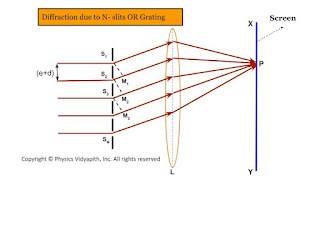Diffraction due to N- slits OR Grating

Theory→

Since plane diffraction grating is an $N$-slit arrangement, the deflection pattern due to it will be the combined diffraction effect of all such slits. Let a plane wavefront of monochromatic light be incident normally on the $N$-parallel slit of the gratings. Each point within the slits then sends out secondary wavelets in all directions.

Let $e$ be the width of each slipped and $d$ be the separation between any two consecutive slits then $(e+d)$ is known as the grating element. The diffracted ray from each slit, then $(e+d)$ is knowns as the grating element. The diffracted ray from each slit is focussed at a point $P$ on the screen $XY$ with the help of a convex lens $L$.

Expression for Intensity→

Let $S_{1}, S_{2}, S_{3},.......$ be the middle point of each slit and $S_{1}M_{1}, S_{2}M_{2}, S_{3}M_{3}, ........S_{N-1}M_{N-1}$ be the perpendicular drawn as shown in the figure.The waves diffracted from each slit is equivalent to a single wave amplitude:

$R=\frac{A\:sin\alpha}{\alpha} \qquad(1)$

The path difference between the waves from slit $S_{1}$ and $S_{2}$ is

$S_{2}M_{1}=(e+d)sin\theta$

The path difference between the waves from slit $S_{2}$ and $S_{3}$ is

$S_{3}M_{2}=(e+d)sin\theta$

The path difference between the waves from slit $S_{n-1}$ and $S_{n}$ is

$S_{N}M_{N-1}=(e+d)sin\theta$

Thus, it is obvious that the path difference between all the consecutive waves is the same and equal to $(e+d)sin\theta$

The corresponding phase difference

$\Delta \phi=\frac{2\pi}{\lambda}(e+d)sin\theta \qquad(2)$

Let $\Delta \phi=2\beta$

$\beta=\frac{\pi}{\lambda}(e+d)sin\theta \qquad(3)$

Thus, the resultant amplitude at $P$ is the resultant amplitude of $N$ waves, each of amplitude $R$ and its common phase difference is $(2\beta)$

$R'=\frac{R \: sin \left( \frac{2N\beta}{2} \right) }{sin \left( \frac{2\beta}{2} \right)} \qquad(4)$

The resultant amplitude at $P$

$R'=\frac{R \: sin N\beta}{sin \beta}$

Where $R=\frac{A\: sin\alpha}{\alpha}$. Now substitute the value of $R$ in above equation and we get

$R'= \frac{A\: sin\alpha}{\alpha} \frac{ \: sin N\beta}{sin \beta} \qquad(5)$

The resultant intensity at $P$

$I=R'^{2}$

 $I=\frac{A^{2} \: sin^{2} \alpha}{\alpha^{2}} \frac{ \: sin^{2} N\beta}{sin^{2} \beta} \qquad(6)$

The factor $\frac{A^{2} \: sin^{2} \alpha}{\alpha^{2}}$ gives the inetnsity pattern due to diffraction from a single slit while the factor $\frac{ \: sin^{2} N\beta}{sin^{2} \beta}$ gives the distribution of intensity due to interference from all the $N$-slit

Principle Maxima→

The intensity will be maximum when $sin\beta=0$ or $\beta=\pm n\pi$

Where $n=0,1,2,3,.....$

But under this condition $sinN\beta$ is also equal to zero. Hence term $\frac{sin N\beta}{sin \beta}$ can be solve by

$\lim_{\beta \rightarrow \pm n\pi} \frac{ \: sin N\beta}{sin \beta}=\lim_{\beta \rightarrow \pm n\pi} \frac{\frac{d}{d\beta} (sin N\beta)}{\frac{d}{d\beta}(sin \beta)}$

$\lim_{\beta \rightarrow \pm n\pi} \frac{ \: sin N\beta}{sin \beta}=\lim_{\beta \rightarrow \pm n\pi} \frac{N cos N\beta}{cos \beta}$

$\lim_{\beta \rightarrow \pm n\pi} \frac{ \: sin N\beta}{sin \beta}=N \lim_{\beta \rightarrow \pm n\pi} \frac{ cos N\beta}{cos \beta}$

Where the value of $\lim_{\beta \rightarrow \pm n\pi} \frac{ cos N\beta}{cos \beta}=1$

$\lim_{\beta \rightarrow \pm n\pi} \frac{ \: sin N\beta}{sin \beta}=N \qquad(7)$

So the maximum intensity

$I=\frac{A^{2} \: sin^{2} \alpha}{\alpha^{2}} N^{2} \qquad(8)$

Thus, the condition for principle maxima

$sin \beta=0$

$\beta=\pm n\pi$

 $(e+d)sin\theta=\pm n \lambda \qquad(9)$

For $n=0$, we get $\theta=0$ This $\theta=0$ gives the direction of zero-order principal maxima. For the value of $n=1,2,3,......$, gives the direction of first, second, third,....... order principal maxima.

Minima →

The intensity will be minimum, when $sin N\beta=0$ but $sin\beta=0$

$N\beta=\pm m\pi$

 $N(e+d)sin\theta=\pm m \lambda \qquad(10)$

Where $m$ can take all integral values except $0,N,2N,3N,......$ because for these values of $m$, $sin\beta=0$ which gives the position of principal maxima.

Secondary maxima→

It is obvious from the above condition of minima, there are $(N-1)$ minima between two successive principal maxima. Hence, there are $(N-2)$ other maxima with alternative minima between two successive principal maxima. These $(N-2)$ maxima are called secondary maxima. To find the condition of secondary maxima equation $(6)$ is differentiated with respect to $\beta$ and equated to zero.

$\frac{dI}{d\beta}= \frac{A^{2}\:sin^{2}\alpha}{\alpha^{2}}2 \frac{sinN\beta}{sin\beta} \left [\frac{sin\beta . N. cosN\beta-sinN\beta . cos\beta}{sin^{2}\beta} \right ]$

$0= \frac{A^{2}\:sin^{2}\alpha}{\alpha^{2}}2 \frac{sinN\beta}{sin\beta} \left [\frac{sin\beta . N. cosN\beta-sinN\beta . cos\beta}{sin^{2}\beta} \right ]$

$N.sin\beta . cosN\beta - sinN \beta . cos\beta=0$

$\tan N\beta = N tan \beta \qquad(11)$

Now construct a right-angled triangle with the sides according to above equation$(11)$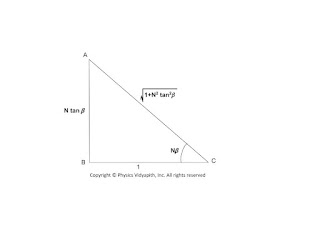Right-angled Triangle for Intensity Calculation
From above triangle:

$sinN\beta=\frac{N tan\beta}{\sqrt{1+N^{2}tan^{2}\beta}} \qquad(12)$

Substituting the value of $sinN\beta$ from the above equation to equation (6)

$I=\frac{A^{2} \: sin^{2} \alpha}{\alpha^{2}} \frac{N^{2} tan^{2}\beta}{1+N^{2}tan^{2}\beta} \frac{ 1}{sin^{2} \beta}$

$I=\frac{A^{2} \: sin^{2} \alpha}{\alpha^{2}} \frac{N^{2}}{1+N^{2}tan^{2}\beta} \frac{ 1}{cos^{2} \beta} \qquad \left(\because tan\beta =\frac{sin\beta}{cos\beta} \right)$

$I=\frac{A^{2} \: sin^{2} \alpha}{\alpha^{2}} \frac{N^{2}}{cos^{2} \beta+N^{2}sin^{2}\beta}$

$I=\frac{A^{2} \: sin^{2} \alpha}{\alpha^{2}} \frac{N^{2}}{1- sin^{2} \beta+N^{2}sin^{2}\beta}$

$I=\frac{A^{2} \: sin^{2} \alpha}{\alpha^{2}} \frac{N^{2}}{1+(N^{2}-1) sin^{2} \beta} \qquad(12)$

Now divide the equation $(12)$ by equation $(8)$ so

 $\frac{Intensity\: of\:secondary\:maxima}{Intensity\:of\:principal\:maxima}=\frac{1}{1+(N^{2}-1) sin^{2} \beta}$

It is obvious from the above equation that When $N$ increases then the intensity of secondary maxima decreases.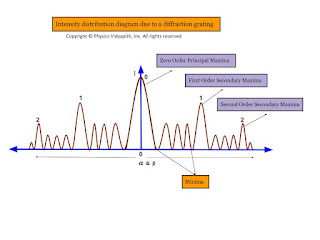Intensity distribution diagram due to a diffraction grating

## Fraunhofer diffraction due to a double slit

Let a plane wavefront be incident normally on slit $S_{1}$ and $S_{2}$ of equal $e$ and separated by an opaque distance $d$.The diffracted light is focused on the screen $XY$. The diffracted pattern on the screen consists of equally spaced bright and dark fringe due to interference of light from both the slits and modulated by diffraction pattern from individual slits.

The diffraction pattern due to double-slit can be explained considering the following points →
• All the points in slits $S_{1}$ and $S_{2}$ will send secondary waves in all directions.

• All the secondary waves moving along the incident wave will be focussed at $P$ and the diffracted waves will be focussed at $P'$

• The amplitude at $P'$ is the resultant from two slit each of amplitude $R=\frac{A\:sin\alpha}{\alpha}$

• T two waves from two-slit $S_{1}$ and $S_{2}$ will interfere at $P'$

•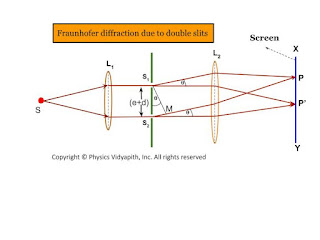Fraunhofer diffraction due to double slits

Expression for Intensity →

$\Delta = S_{2}M$

$\Delta=(e+d)sin\theta\qquad(1)$

The corresponding phase difference →

$\Delta\phi= \frac{2\pi}{\lambda}(e+d)sin\theta \qquad(2)$

Let $\Delta \phi =2 \beta \qquad(3)$

$\beta=\frac{\pi}{\lambda}(e+d)sin\theta\qquad(4)$

The resultant amplitude at $P'$ can be obtained by the vector addition method. The resultant amplitude at $P'$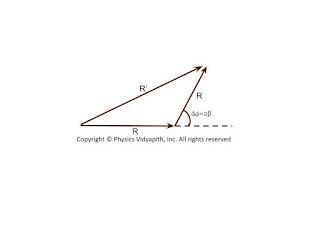Resultant Vector
$R'^{2}=R^{2}+R^{2}+2R.R.cos\Delta\phi$

$R'^{2}=R^{2}+R^{2}+2R.R.cos2\beta$ {\qquad $\because 2\beta=\Delta\phi$}

$R'^{2}=2R^{2}+2R^{2}cos2\beta$

$R'^{2}=2R^{2} \left( 1+cos2\beta \right)$

$R'^{2}=4R^{2} cos^{2}\beta \qquad(5)$

Where $R$ - Resultant amplitude of each slit $S_{1}$

$R=\frac{A\: sin\alpha}{\alpha} \qquad(6)$

Substituting the value of $R$ in equation $(5)$

$R'^{2}=4 A^{2} \frac{sin^{2}\alpha}{\alpha^{2}} cos^{2} \beta \qquad(7)$

 $R'=2 A \frac{sin\alpha}{\alpha} cos\beta \qquad(8)$

The intensity of the resultant diffraction pattern at $P'$

 $I=4 A^{2} \frac{sin^{2}\alpha}{\alpha^{2}} cos^{2} \beta \qquad(9)$

Where $\alpha=\frac{\pi}{\lambda}e\:sin\theta \qquad(10)$

The resultant intensity at any point is the contribution of the following two factors →

• The factor $\frac{A^{2}sin^{2}\alpha}{\alpha^{2}}$, represents the intensity distribution due to diffraction from any individual slits.

• The factor $cos^{2}\beta$ represents the intensity distribution due to interference of waves from two parallel slits.

• Condition for Maxima and Minima →
1. Maxima and minima due to diffraction term
2. Maxima and minima due to interference term

1. Maxima and minima due to diffraction term →

i.) Principal Maxima →

The diffraction term $\frac{A^{2}sin^{2}\alpha}{\alpha^{2}}$ gives the central maxima, for $\alpha=0$ so

 $\frac{\pi}{\lambda}e\: sin\theta=0$

$sin\theta =0$

 $\theta=0$

ii.) Minima →

The diffraction term $\frac{A^{2}sin^{2}\alpha}{\alpha^{2}}$ gives the central minima, for $sin\alpha=0$ so

$\alpha=\pm m\pi$

$e\:sin\theta=\pm m\pi$

iii.) Secondary Maxima →

The secondary maxima are obtained in the direction given by →

 $\alpha= \pm\frac{3\pi}{2},\pm\frac{5\pi}{2},\pm\frac{7\pi}{2},..............$

2. Maxima and minima due to interference term →

i.) Maxima

The interference term $cos^{2}\beta$ gives maxima in the direction →

$cos^{2}=1$

$\beta=\pm n \pi$

$\frac{\pi}{\lambda}(e+d)sin\theta= \pm n \pi$

$(e+d)sin\theta= \pm n \lambda$

Where $n=0,1,2,3,.....$

In the direction $\theta=0\circ$, the principle maxima due to interference and diffraction coincide.

ii.) Minima

The interference term $cos^{2}\beta$ gives minima in the direction →

$cos^{2}\beta=0$

$\beta=\pm(2n+1)\frac{\pi}{2}$

 $(e+d)sin\theta=\pm(2n+1)\frac{\pi}{2}$

The intensity distribution curve due to the diffraction term, interference term, and the combined effect is shown in the figure below →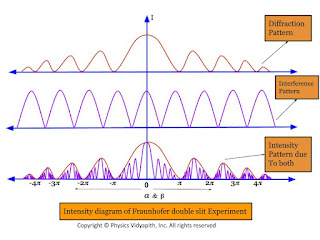Intensity diagram of Fraunhofer double slit Experiment

## Dispersive power of plane diffraction grating and its expression

Dispersive power of plane diffraction grating:

The dispersive power of a diffraction grating is defined as:

The rate of change of the angle of diffraction with the change in the wavelength of light are called dispersive power of plane grating.

If the wavelenght changes from $\lambda$ to $\lambda +d\lambda$ and respective change in the angle of diffraction be from $\theta$ to $\theta+d\theta$ then the ratio $\left(\frac{d\theta}{d\lambda} \right)$

Expression of Dispersive power of a plane diffraction grating:

The grating equation for a plane transmission grating for normal incidence is given by

$(e+d)sin\theta=n\lambda \qquad(1)$

Where$(e+d)$ - Grating Element$\qquad \:\: \theta$ - Diffraction angle for spectrum of $n^{th}$ order

Differentiating equation $(1)$ with respect to $\lambda$, we have

$(e+d)cos\theta \left( \frac{d\theta}{d\lambda} \right)=n$

$\frac{d\theta}{d\lambda}=\frac{n}{(e+d)cos\theta}$

$\frac{d\theta}{d\lambda}=\frac{n}{(e+d)\sqrt{1-sin^{2}\theta}} \qquad(2)$

Now substitute the value of $sin\theta$ from equation$(1)$ in equation$(2)$

$\frac{d\theta}{d\lambda}=\frac{n}{(e+d)\sqrt{1- \frac{n^{2}\lambda^{2}}{(e+d)^{2}}}}$

 $\frac{d\theta}{d\lambda}=\frac{1}{\sqrt{\left(\frac{e+d}{n} \right)^{2}}- \lambda^{2}}$

Here $d\theta$- Angular separation between two lines

The above equation gives the following conclusions:

• The dispersive power is directly proportional to the order of spectrum$(n)$

• The dispersive power is inversely proportional to the grating element $(e+d)$.

• The dispersive power is inversely proportional to the $cos\theta$ i.e Larger value of $\theta$, higher is the dispersive power.

• ## Fraunhofer diffraction due to a single slit

Let $S$ is a point monochromatic source of light of wavelength $\lambda$ placed at the focus of collimating lens $L_{1}$. The light beam is incident normally from $S$ on a narrow slit $AB$ of width $e$ and is diffracted from it. The diffracted beam is focused at the screen $XY$ by another converging lens $L_{2}$. The diffraction pattern having a central bright band followed by an alternative dark and bright band of decreasing intensity on both sides is obtained.

Analytical Explanation:

Light from the source $S$ is incident as a plane wavefront on the slit $AB$. According to Huygen's wave theory, every point in $AB$ sends out secondary waves in all directions. The undeviated ray from $AB$ is focused at $C$ on the screen by the lens $L_{2}$ while the rays diffracted through an angle $\theta$ are focussed at point $p$ on the screen. The rays from the ends $A$ and $B$ reach $C$ in the same phase and hence the intensity is maximum.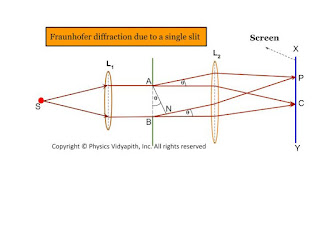Fraunhofer diffraction due to a single slit

Expression for intensity:

To find the intensity at $P$, Let us draw normal $AN$ on $BN$. Therefore the path difference between the extreme rays is

$\Delta=BN$

$\Delta=AB \: sin \theta$

$\Delta=e \: sin \theta \qquad(1)$

Where $AB=e$ (width of the slit)

The phase difference

$\Delta \phi =\frac{2 \pi}{\lambda} e \: sin\theta \qquad(2)$ {from eq$(2)$}

Let AB is divided into a large number $n$ of equal parts then there may be infinitely large number of the point sources of secondary wavelets between $A$ and $B$. The phase difference between any two consecutive parts is, therefore

$\frac{1}{n} \Delta \phi=\frac{1}{n} \frac{2 \pi}{\lambda} e \: sin\theta$

According to the theory of the composition of $n$ vibration each of amplitude $a$ and common phase difference, $\delta$ between successive vibrations, the resultant amplitude at $P$ is given by

$R=a\frac{sin(\frac{n\delta}{2})}{sin(\frac{\delta}{2})} \qquad(4)$

Put the value of $\delta$ in equation $(4)$ so

$R=a \frac{sin [(\frac{1}{n} \frac{2\pi}{\lambda}e\: sin \theta) \frac{n}{2}]}{sin [\frac{1}{2}(\frac{1}{n} \frac{2\pi}{\lambda}e\: sin \theta)]}$

$R=a \frac{sin (\frac{\pi}{\lambda}e\: sin \theta)}{sin [\frac{1}{n} (\frac{\pi}{\lambda}e\: sin \theta)]} \qquad(5)$

Let $\alpha=\frac{\pi}{\lambda}e\: sin \theta \qquad(6)$

Then from equation $(5)$

$R=a \frac{sin \alpha }{sin (\frac{\alpha}{n})} \qquad(7)$

For the large value of $n$, the value of $\frac{\alpha}{n}$ is very small, Therefore $sin \alpha \approx \frac{\alpha}{n}$

Then equation $(7)$ can be written as

$R=a \frac{sin \alpha}{\frac{\alpha}{n}}$

$R=\frac{na \: sin\alpha}{\alpha}$

$R=\frac{A \: sin\alpha}{\alpha} \qquad(8)$

Where $A$ is total amplitude of $n$ vibration.$R$ is resultant amplitude of $n$ vibration

So the resultant intensity at $P$

$I=R^{2}$

$I=\frac{A^{2} \: sin^{2}\alpha}{\alpha^{2}} \qquad(9)$

Condition for Principle Maxima:

From equation $(7)$

$R=\frac{A \: sin\alpha}{\alpha}$

$R=\frac{A}{\alpha} [\alpha - \frac{\alpha^{3}}{3!} + \frac{\alpha^{5}}{5!} -\frac{\alpha^{7}}{7!} + ..... ]$

$R=A [1 - \frac{\alpha^{2}}{3!} + \frac{\alpha^{4}}{5!} -\frac{\alpha^{6}}{7!} + .....]$

If $\alpha=0$ then resultant amplitude will be maximum then

 $R=A$

And

$\frac{\pi}{\lambda}e\: sin \theta = 0$ {From equation $(6)$}

$sin \theta = 0$

$sin \theta = sin 0^{\circ}$

 $\theta = 0^{\circ}$

The resultant intensity at $P$ will be the maximum. For $\theta=0^{\circ}$ and called the principal maxima. Hence the intensity of the principal maxima:

 $I_{0}=A^{2}$

Condition for Minima:

It is clear for from equation $(8)$ thqat the intensity will be minimum when $sin\alpha=0$ but $\alpha \neq 0$ So.

$sin \alpha =0$

$sin \alpha= sin (m \pi)$

 $\alpha=\pm m \pi$

And

$\frac{\pi}{\lambda}e\: sin \theta = \pm m \pi$ {From equation $(6)$}

 $\frac{\pi}{\lambda}e\: sin \theta = \pm m \pi$

The value of $m=1,2,3,4,5,......$ gives the direction of first, second,third,.....minima.

Condition for Secondary Maxima: In the diffraction pattern, there are secondary maxima in addition to principal maxima. The condition of secondary maxima may be obtained by differentiating equation $(9)$ with respect to $\alpha$ and equating it to zero. Hence

$\frac{dI}{d\alpha}=\frac{d}{d\alpha} \left( A^{2} \frac{sin^{2}\alpha}{\alpha^{2}}\right)$

$\frac{dI}{d\alpha}=A^{2} 2 \left(\frac{sin\alpha}{\alpha} \left [\frac{\alpha \: cos\alpha - sin\alpha}{\alpha^{2}} \right ] \right)$

But for maxima $\frac{dI}{d\alpha}=0$

So $A^{2} 2 \left(\frac{sin\alpha}{\alpha} \left [\frac{\alpha \: cos\alpha - sin\alpha}{\alpha^{2}}\right ] \right)=0$

$\frac{\alpha \: cos\alpha - sin\alpha}{\alpha^{2}}=0$

$\alpha \: cos\alpha - sin\alpha=0$

$\alpha=tan\alpha$

The above equation can be solved graphically by plotting the curves

$y=\alpha$

$y=tan\alpha$

The equation $y=\alpha$ gives the straight line passing through the origin and making an angle $45^{\circ}$ with the x-axis.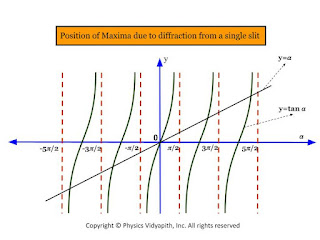Position of maxima due to diffraction from a single slit

The pont of intersection of these two curves gives the value of $\alpha$ satisfying the equation $\alpha=tan\alpha$. These points correspond to the value of

 $\alpha= 0, \frac{\pm 3\pi}{2}, \frac{\pm 5\pi}{2}, \frac{\pm 7\pi}{2}, ................$

The first value $\alpha=0$, gives the position of principle maxima while the value of $\alpha= \frac{\pm 3\pi}{2}, \frac{\pm 5\pi}{2}, \frac{\pm 7\pi}{2}, ................$ gives the position first secondary maxima, second secondary maxima, and third secondary maxima and so on respectively.

The intensity of the first secondary maxima

$I_{1}=A^{2} \left [\frac{sin \left(\frac{3\pi}{2} \right)}{\frac{3\pi}{2}} \right ]^{2}$

 $I_{1}=\frac{4}{9\pi^{2}}A^{2}=\frac{A^{2}}{22}=\frac{I_{0}}{22}$

Similarly, the intensities of secondary maxima

$I_{2}=A^{2} \left [\frac{sin \left(\frac{5\pi}{2} \right)}{\frac{5\pi}{2}} \right ]^{2}$

 $I_{2}=\frac{4}{25\pi^{2}}A^{2}=\frac{A^{2}}{62}=\frac{I_{0}}{62}$

Thus, It is obvious from the value of $I_{0}, I_{1}, I_{2},.....$, etc that the diffraction pattern consists of a bright central maximum followed by minima of zero intensity and then secondary maxima of decreasing intensity on either side of it.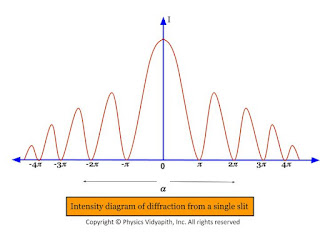Intensity diagram of diffraction from a single slit

It is also obvious from the value of $I_{0}, I_{1}, I_{2},.....$, etc that the relative intensities of successive maxima are nearly

 $I_{0}: I_{1}: I_{2}:.....=1:\frac{4}{9\pi^{2}}: \frac{4}{25\pi^{2}}: \frac{4}{49\pi^{2}}:.......$

## Interference of light and classification of Interference

Interference of light:

When two or more waves, having the same frequency and constant phase difference, travel simultaneously in the same region of a medium, these waves superimpose on each other and a resultant wave is obtained which has intensity at some points maximum and some points minimum in the region. This is phenomenon is known as interference of light.

When the intensity of the resultant wave is maximum in the region then this is called constructive interference.

when the intensity of the resultant wave is minimum in the region then this is called destructive interference.

Classification of Interference:

The phenomenon of interference may be grouped into two categories depending upon the formation of two coherent sources in practice.The interference of light is classified into two categories:

• Division of amplitude

• Division of wavefront

• Division of amplitude:

In this method, the amplitude of the incident beam is divided into two or more parts are either by partial reflection or refraction. the beams travel in different paths, are superimposed on each other, and form the interference pattern.

Example: Interference in the thin film, Newton's rings, and Michelson's interferometer are examples of two-beam interference and Fabry-Perot interferometer is the example of multiple-beam interference.

Division of Wavefront:

Under this category, the coherent sources are obtained by dividing the wavefront, originating from a common source, by employing mirrors, biprisms, or lenses. This class of interference requires initially a point source or a narrow slit source. The instruments used to obtain coherent sources and hence interference by division of wavefront are fresnel biprism fresnel Mila mirror Lloyd's mirror laser etc.

## Newton's rings in reflected monochromatic light

Newton's Rings:

Experimental Arrangement:

• A plano-convex lens $l_{1}$ of large radius of curvature is placed on a plane glass plate $G_{1}$ with the curved surface touching the glass plate.

• An air film is enclosed between the curved surface of the lens and the glass plate.

• A sodium vapor lamp $S$ is kept at the focus of a biconvex lens $L$ which converts the diverging beam of light into a parallel beam. The parallel beam of light is made to fall on a glass plate $G_{2}$ kept at an angle of $45^{\circ}$ with the incident beam.

• A part of incident light is reflected toward the plano-convex lens. This light is again reflected back, partially from the top and partially from the bottom of the air fil and transmitted by the glass plate $G_{2}$. The interference of these rays is observed through a microscope $M$.

•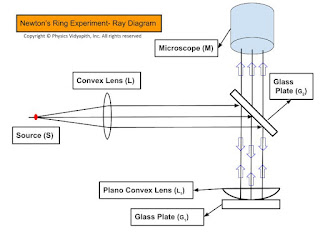Newtons Rings Experiment Setup-Ray Diagram

Explanation of the formation of Newton's rings:

• Division of amplitude takes place at the curved surface of the plano-convex lens.

• The incident light is partially reflected and partially transmitted at the curved surface.

• The transmitted ray is reflected from the glass plate. These two rays interfere in reflected light

• The path difference between these rays depends on the thickness of the air film enclosed between the curved surfaces of the lens and glass plate which increases radially outward from the center. The thickness of the air film is zero at the center

•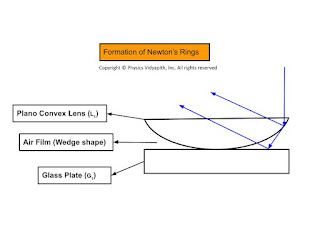Formation of Newton Rings

Path difference:

Let $t$ is the thickness of air film at a radial distance of $r$ and $R$ is the radius of curvature. So the path difference for a wedge-shaped film is

$\Delta=2 \mu t \: cos(r+\alpha) \pm \frac{\lambda}{2} \qquad(1)$

HereRefractive index of air film ($\mu)=1$$\alpha$ is very small i.e. $\alpha \approx 0$For normal incidence $r=0$ i.e $cos(\alpha+r)=1$

Now apply the above condition to equation $(1)$ then we get

$\Delta = 2t + \frac{\lambda}{2} \qquad(2)$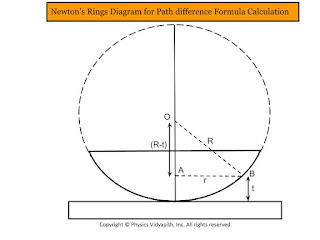Newton’s Rings Diagram for Path difference Formula Calculation

Form figure, In $\Delta OAB$, apply the Pythagoras theorem

$OA^{2}=OB^{2}+AB^{2}$

$R^{2}=(R-t)^{2}+r^{2}$

$R^{2}=R^{2}+t^{2}-2Rt+r^{2}$

$2Rt=t^{2}+r^{2}$

As $t$ is very small, $t^{2}$ can be neglected in comparison with $r^{2}$. So

$2Rt=r^{2}$

$2t=\frac{r^{2}}{R} \qquad(3)$

Here $r$- Radius of a circle for which thickness is $t$

If $D$ is the diameter of this circle then

$r=\frac{D}{2}$

So from equation $(3)$

$2t=\frac{D^{2}}{4R} \qquad(4)$

Substitute the value of $2t$ in equation $(2)$ then we get

 $\Delta = \frac{D^{2}}{4R}+\frac{\lambda}{2}$

Diameter of Bright rings:

For bright rings, the condition for constructive interference is satisfied. i.e.

$\Delta =n \lambda$

So from the above equations, we get

$\frac{D^{2}}{4R}+\frac{\lambda}{2}=n \lambda$

Where $D_{n}$- Diameter of the $n^{th}$ bright ring

$\frac{D^{2}}{4R}=(2n-1) \frac{\lambda}{2}$

 $D_{n}= \sqrt {2 \lambda R (2n-1)}$

Where $n=1,2,3,4........$

 $D_{n} \propto \sqrt{(2n-1)}$

i.e The diameter of the bright rings are proportional to the square root of odd natural numbers.

Diameter of Dark ring:

Condition for destructive interference is satisfied for dark rings i.e.

$\Delta=(2n+1)\frac{\lambda}{2}$

$\frac{D^{2}}{4R}+\frac{\lambda}{2}=(2n+1)\frac{\lambda}{2}$

$\frac{D^{2}}{4R}+\frac{\lambda}{2}=n \lambda + \frac{\lambda}{2}$

$\frac{D^{2}}{4R}=n \lambda$

 $D_{n}= \sqrt{4Rn\lambda}$

 $D_{n} \propto \sqrt{n}$

Where $n=1,2,3,4........$

i.e The diameter of dark rings are proportional to square root of natural numbers.

## Fringe width of wedge shaped thin film for normal incidence

Derivation of Fringe width of the wedge-shaped thin film:
• The distance between two consecutive bright (or dark) fringes is called the fringe width.

• If the $n^{th}$ bright fringe is formed at a distance $x_{n}$ from the edge of the wedge shaped film where the thickness is $t_{n}$. So the path difference for $x_{n}$ bright fringe:

• $(2n-1) \frac{\lambda}{2}= 2 \mu \: t_{n} \: cos(\alpha+r) \qquad(1)$

For Normal IncidenceThe incident Angle $i=0$The refracted Angle $r=0$

then from equation $(1)$

$(2n-1) \frac{\lambda}{2}= 2 \mu \: t_{n} \: cos\alpha \qquad(2)$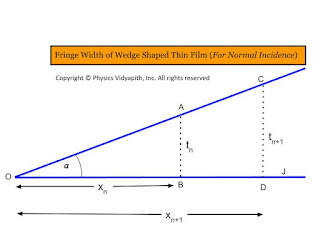Fringe width of wedge-shaped thin film for normal incidence

From the figure, In $\Delta OAB$

$tan \alpha = \frac{t_{n}}{x_{n}}$

$t_{n}=x_{n} \: tan \alpha \qquad(3)$

Now put the value of $t_{n}$ in equation $(2)$

$(2n-1) \frac{\lambda}{2}= 2 \mu \: x_{n} \: tan \alpha \: cos\alpha$

$(2n-1) \frac{\lambda}{2}= 2 \mu \: x_{n}\: \frac{sin \alpha}{cos\alpha} \: cos\alpha$

$(2n-1) \frac{\lambda}{2}= 2 \mu \: x_{n} \: sin \alpha \qquad(4)$

Similarly for $(n+1)^{th}$ bright fringe i.e put the $(n+1)$ in place of $n$ in above equation then we get

$\left\{ 2(n+1)-1 \right\} \frac{\lambda}{2}= 2 \mu \: x_{n+1} \: sin \alpha$

$(2n+1) \frac{\lambda}{2}= 2 \mu \: x_{n+1} \: sin \alpha \qquad(5)$

Subtract the equation $(5)$ and equation$(4)$

$2\mu \: (x_{n+1}-x_{n})\: sin \alpha =\lambda$

$2\mu \beta \: sin \alpha =\lambda$

Where $\beta=$ fringe Width$(x_{n+1}-x_{n})$

 $\beta=\frac{\lambda}{2\mu\: sin \alpha}$

If $\alpha$ is very small so $sin \alpha \approx \alpha$

 $\beta=\frac{\lambda}{2\mu\: \alpha}$

## Interference of light due to a wedge shaped thin film

Derivation of interference of light due to a wedge-shaped thin film: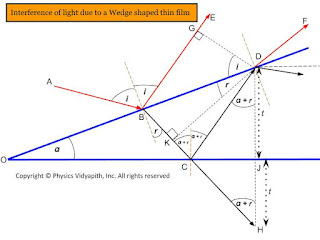Interference of light due to wedge-shaped thin film

The wedge-shaped film is bound by two plane surfaces inclined at angle $\alpha$.

The thickness t of the film varies uniformly from zero at the edge to its maximum value at the other end.

A Ray of light ab incident on the film will be partially reflected along be and partially transmitted along $BC$. The ray $BC$ will be partially reflected along $CD$ which will be again partially transmitted along $BF$. The two rays $BE$ and $DF$ in reflected light diverge.

The path difference between ray $BE$ and $DF$ is

$\Delta=\mu(BC+CD)-BG \qquad(1)$

Where $\mu$- Refractive index of the film

$\Delta CDJ$ and $\Delta CHJ$ are congruent so

$\begin{Bmatrix} CD=CH \\ DJ=JH=t \end{Bmatrix} \qquad(2)$

From equation $(1)$

$\Delta=\mu(BK+KC+CH)-BG \qquad (\because BD=BK+KC)$

$\Delta=\mu(BK+KH)-BG \qquad (3)$

In $\Delta BDG$-

$sin\:i = \frac{BG}{BD}$

$BG=BD sin \: i \qquad(4)$

In $\Delta BDK$

sin\:r =\frac{BK}{BD}

$BD=\frac{BK}{sin \: r} \qquad(5)$

From equation $(4)$ and equation $(5)$-

$BG=BK. \frac{sin \: i}{sin \: r}$

$BG=\mu . BK \qquad(6) \qquad (\because \mu=\frac{sin \: i}{sin \: r})$

Now put the value of $BG$ in equation $(3)$

$\Delta= \mu(BK+KH)- \mu. BK$

$\Delta= \mu.KH \qquad(7)$

In $\Delta DKH$

$cos \: (\alpha + r)= \frac{KH}{DH}$

$KH=DH \: cos \: (\alpha + r)$

$KH=(DJ+JH) \: cos \: (\alpha + r)$

$KH=2t \: cos \: (\alpha + r) \qquad(8)$ {From equation $(2)$}

Now put the value of $KH$ in equation $(7)$

 $\Delta= 2\mu t \: cos \: (\alpha + r)$

The ray $DF$ suffers a phase change of $\pi$ due to reflection from a denser medium at $C$. Whereas there is not any change in phase due to reflection for ray $BE$.

 $\Delta= 2\mu t \: cos \: (\alpha + r) \pm \frac{\lambda}{2}$

For Constructive Interference:

$\Delta= n \lambda$

$\Delta= 2\mu t \: cos \: (\alpha + r) \pm \frac{\lambda}{2}= n \lambda$

 $2\mu t \: cos \: (\alpha + r)= (2n \pm 1) \frac{\lambda}{2}$

For Destructive Interference:

$\Delta= (2n \pm 1) \frac{\lambda}{2}$

$\Delta= 2\mu t \: cos \: (\alpha + r) \pm \frac{\lambda}{2}=(2n \pm 1) \frac{\lambda}{2}$

 $\Delta= 2\mu t \: cos \: (\alpha + r) =2n \lambda$

## Interference of light due to thin film

Derivation of interference of light due to thin-film: Let's consider a Ray of light $AB$ incident on a thin film of thickness $t$ and the refractive index of a thin film is $\mu$

The ray $AB$ is partially reflected and partially transmitted at $B$. The transmitted BC is against partially transmitted and partially reflected at $C$. The reflected ray $CD$ is partially reflected and partially refracted at $D$.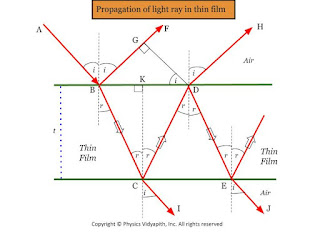Propagation of light ray in thin film
The interference pattern in reflected light will be due to ray $BF$ and $DH$ which are coherent as they are both derived from the same Ray $AB$.

The interference pattern in transmitted light will be due to ray $CI$ and $EJ$.

The path difference between $BF$ and $DH$ ray will be

$\Delta=\mu(BC+CD)-BG \qquad(1)$

The triangle $\Delta BCK$ and $\Delta CDK$ are congruent because

$\begin{Bmatrix} BK=KD \\ BC=CD \\ CK=t \end{Bmatrix} \qquad (2)$

From equation $(1)$ and $(2)$

$\Delta=2 \mu BC-BG \qquad(3)$

In $\Delta BCK$

$cos \: r= \frac{CK}{BC}$

$BC= \frac{t}{cos \: r} \qquad(4)$

In $\Delta BGD$

$sin \: i= \frac{BG}{BD}$

$BG= BD \: sin\: i$

$BG= (BK+KD)\: sin\: i$

$BG= 2BK \: sin\: i \qquad(5)$ {From eqaution $(2)$}

Again In $\Delta BCK$

$tan \:r = \frac{BK}{CK}$

$BK=t.tan\:r \qquad(6)$

Put the value of $BK$ in equation $(5)$

$BG=2t\:sin \:i .tan\: r$

$BG=2t\:sin \:i .\frac{sin \: r}{cos \: r}$

$BG= 2t \frac{sin \:i}{sin \: r} \frac{sin^{2} \:r}{cos \: r}$

$BG=2\mu t \frac{sin^{2} \:r}{cos \: r} \qquad (7)\qquad \left( \mu =\frac{sin \:i}{sin \: r}\right)$

Now put the value of $BG$ and $BC$ in equation $(3)$

$\Delta= \frac{2 \mu t}{cos \: r}-2\mu t\frac{sin^{2} \:r}{cos \: r}$

$\Delta=\frac{2 \mu t}{cos \:r} \left[1-sin^{2} \:r \right]$

$\Delta=2 \mu t \frac{cos^{2} \:r}{cos \:r}$

 $\Delta=2 \mu t \: cos \:r$

Interference in a reflected ray:

The ray $BF$ suffers phase change of $\pi$ due to reflection from the denser medium at $B$. Therefore the path difference of $\frac{\lambda}{2}$ is introduced between two rays due to reflection-

 $\Delta= 2\mu t \: cos\:r \pm \frac{\lambda}{2}$

Constructive Interference due reflected ray:

for constructive interference

$\Delta=n \lambda$

so from the above equations, we get

$2\mu t \: cos\: r \pm \frac{\lambda}{2}=n \lambda$

 $2\mu t \: cos\: r = (2n \pm 1)\frac{\lambda}{2}$

Destructive Interference due to reflected ray:

for destructive interference

$\Delta=(2n \pm 1)\frac{\lambda}{2}$

so from the above equations, we get

$2\mu t \: cos\: r \pm \frac{\lambda}{2}=(2n \pm 1)\frac{\lambda}{2}$

 $2\mu t \: cos\: r =n \lambda$

Interference in transmitted ray:

The ray $CI$ and $EJ$ in transmitted Ray have the same path difference as a reflected ray. There is not any change in phase for $CI$ due to reflection as it gets transmitted at $C$. The ray $EJ$ also does not undergo phase change due to reflection as it is reflected from the rarer medium at $C$ and $D$.

$\Delta= 2\mu t \: cos\:r$

Constructive Interference due to transmitted ray:

for constructive interference

$\Delta=n \lambda$

so from the above equations, we get

 $2\mu t \: cos\: r =n \lambda$

Destructive Interference due to transmitted ray:

for destructive interference

$\Delta=(2n \pm 1)\frac{\lambda}{2}$

so from the above equations, we get

 $2\mu t \: cos\: r =(2n \pm 1)\frac{\lambda}{2}$

 $2\mu t \: cos\: r =n \lambda$

### Principle, Construction and Working of the Ruby Laser